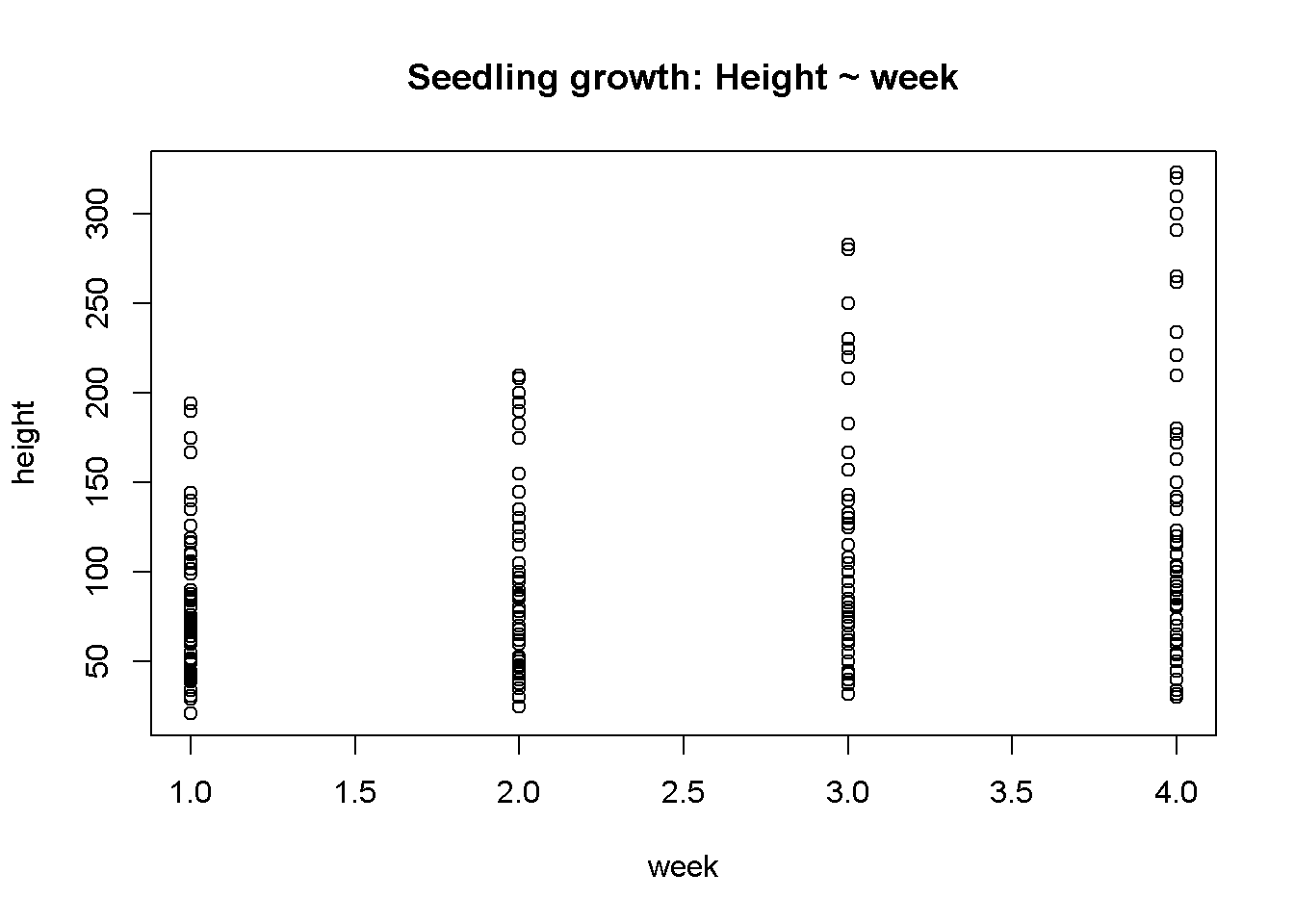Changes to this lab will be announced via email and the course FaceBook page CalU EcoStats.

For more labs and tutorials see my WordPress Site lo.brow.R

1 Outline

• Regression model building
• Regression model testing
• Regression diagnostics
• Transformation
• Reporting results of regression
• Multiple regression
• Repeated measures data

** These data come from:** Meredith et al 1991 Repeated measures experiments in forestry: focus on analysis of response curves. Can. J. For. Res.

2 Data formatting

• Wide format
• Long form

2.1 Wide format

• The 1st column is the concentration of aluminum (AL) that sugar maple seeds were treated with.
• Each ROW is a different tree
• Height growth was then measured for 4 weeks
• Height was recored in a seperate column
• This is called “wide” format b/c data for an individual thing being studied is read left to right
• This is a common way to collect data and present it in a table and is easy to read

The size of the dataframe

dim(dat.orig)
##  67  6

2.1.0.1 Data in wide format

##   X conc.AL ht.wk.1 ht.wk.2 ht.wk.3 ht.wk.4
## 1 1       0      60      62      78     104
## 2 2       0      41      50      60      60
## 3 3       0      85      97     115     120
## 4 4       0      88      87      90      80
## 5 5       0      66      65      80      95
## 6 6       0     106     100     133     172

2.2 Long format

• This is how R needs data to be formatted for regression and ANOVA
• t.test also uses data in this format
• Note that there are MANY rows of data
• Data in this format is not very easy to read by eye
• This format matches how the math gets done by the computer
• ALL respone data (y variables, “height”) are in a SINGLE column
• ALL predictor data (x variable, week) are in a single columns

The size of the dataframe

dim(data.long)
##  268   4

2.2.0.1 Data in long format

##   X height week conc.AL
## 1 1     60    1       0
## 2 2     41    1       0
## 3 3     85    1       0
## 4 4     88    1       0
## 5 5     66    1       0
## 6 6    106    1       0

3 Plotting regression data

• Plot regression style data wit the plot() command
plot(height ~ week,
data = data.long,
main = "Seedling growth: Height ~ week"
)3.0.1 Extra: changing plotting symbols and other features

You can change … * color using col = 2 (or another number) * symbol with pch = … * x axis label w/ xlab = * y axis label w/ ylab =

4 Basic regression analysis 1: height ~ week

• We are going to do a basic regression analysis of these data.
• A VERY important caveat here is that we are ignoring the fact that the same seedlings were measured multiple times
• We are therefore treating each row of data as independent * That is, as if EACH ROW represented a completley different plant that had not been measured before and will not be measured again!
• This is therefore a completely invalid analysis and just done for illustration!
• In reality, this data is considered “repeated measures” or “longitudinal”
• Proper analysis requires calculating averages growth for each plant, using just the final measurement, or using special statistical techniques

4.1 Model fitting

We fill fit a “null” and alternative model.

4.1.1 Fit a null and alternative model

“Null”" Model

model.null <- lm(height ~ 1,
data = data.long)

“Alternative”" Model

model.alt <- lm(height ~ week,
data = data.long)

4.2 Test the models

anova(model.null,
model.alt)
## Analysis of Variance Table
##
## Model 1: height ~ 1
## Model 2: height ~ week
##   Res.Df    RSS Df Sum of Sq      F    Pr(>F)
## 1    267 964926
## 2    266 907135  1     57791 16.946 5.135e-05 ***
## ---
## Signif. codes:  0 '***' 0.001 '**' 0.01 '*' 0.05 '.' 0.1 ' ' 1

The F statistic and p-value are very important quantities that need to be reported in a paper.

TASK: Write the F-statistc and p-value for test on the worksheet.

NOTE: Because we sampled the sample plant multiple tiems this p-value is WAY too small and our sample size is WAY too big. This is a form of psuedoreplication.

4.3 Examine the model against the raw data

par(mfrow = c(1,2))
plot(height ~ week, data = data.long,
main = "Height ~ 1"
)
abline(model.null, col = 2, lwd = 3)

plot(height ~ week,
data = data.long,
main = "Height ~ week"
)
abline(model.alt, col = 2, lwd = 3)